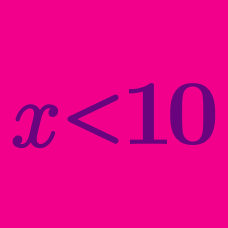Algebra

# Linear Inequalities - Problem Solving

If $x+y = 7$ and $4 \leq x \leq 19,$ what is the range of $y?$

If the inequality $a^2(x+1)>576 x+24a$ holds for all real numbers $x,$ what is the constant $a?$

Let $x>\frac{14}{13}$ be the solution to the inequality $bx-(a+b)<0,$ where $a$ and $b$ are constants. Then what is the solution to the inequality $ax+2a+b<0?$

A factory produces two products $A$ and $B$ using raw materials $p$ and $q$. It uses $3$ units of $p$ and $1$ unit of $q$ to produce one unit of $A$. It uses $1$ unit of $p$ and $2$ units of $q$ to produce one unit of $B$. The maximum daily supplies are $30$ units of $p$ and $20$ units of $q.$ If the profit per unit of $A$ is $\3$ and the profit per unit of $B$ is $\2$, what is the maximum total daily profit (in dollars)?

Real numbers $x$ and $y$ satisfy $-3 < x < 1$ and $-1 < y < 5$. What is the range of $x - y ?$

×